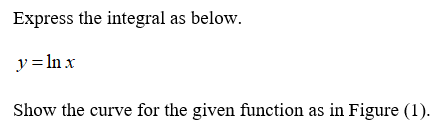# Let R be the “triangular” region in the first quadrant that is bounded above by the line y = 1, below by the curve y = ln x, and on the left by the line x = 1. Find the volume of the solid generated by revolving R about a. the x-axis. b. the line y = 1.

Question
16 views

Let R be the “triangular” region in the first quadrant that is bounded above by the line y = 1, below by the curve y = ln x, and on the left by the line x = 1. Find the volume of the solid generated by revolving R about a. the x-axis. b. the line y = 1.

check_circle

Step 1Step 2

Calculate the volume of the solid.

Step 3

Integrate the abov...

### Want to see the full answer?

See Solution

#### Want to see this answer and more?

Solutions are written by subject experts who are available 24/7. Questions are typically answered within 1 hour.*

See Solution
*Response times may vary by subject and question.
Tagged in
MathCalculus

### Other